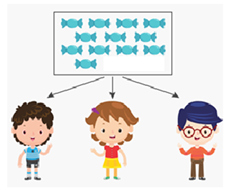Mathematics
Easy

Question

# The candies each child gets is?## 4    3    5    6Hint:

## The correct answer is: 4

### 12 ÷ 3 = 4Each child gets 4 candies.

Let’s count the number of candies in the figure. There are a total of 12 candies. these candies need to be divided equally into 3 kids. Therefore, number of candies each kid gets = 12/3 = 4 candies each. This is a simple division problem.

### Related Questions to study#### With Turito Foundation.#### Get an Expert Advice From Turito.